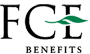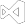Read Excel Files in Core
``````using IronXL;
using System;
using System.Linq;

// Supported for XLSX, XLS, XLSM, XLTX, CSV and TSV

// Select worksheet at index 0
WorkSheet workSheet = workBook.WorkSheets;

// Get any existing worksheet
WorkSheet firstSheet = workBook.DefaultWorkSheet;

// Select a cell and return the converted value
int cellValue = workSheet["A2"].IntValue;

// Read from ranges of cells elegantly.
foreach (var cell in workSheet["A2:A10"])
{
Console.WriteLine("Cell {0} has value '{1}'", cell.AddressString, cell.Text);
}

// Calculate aggregate values such as Min, Max and Sum
decimal sum = workSheet["A2:A10"].Sum();

// Linq compatible
decimal max = workSheet["A2:A10"].Max(c => c.DecimalValue);``````
``````Imports IronXL
Imports System
Imports System.Linq

' Supported for XLSX, XLS, XLSM, XLTX, CSV and TSV
Private workBook As WorkBook = WorkBook.Load("sample.xlsx")

' Select worksheet at index 0
Private workSheet As WorkSheet = workBook.WorkSheets(0)

' Get any existing worksheet
Private firstSheet As WorkSheet = workBook.DefaultWorkSheet

' Select a cell and return the converted value
Private cellValue As Integer = workSheet("A2").IntValue

' Read from ranges of cells elegantly.
For Each cell In workSheet("A2:A10")
Console.WriteLine("Cell {0} has value '{1}'", cell.AddressString, cell.Text)
Next cell

' Calculate aggregate values such as Min, Max and Sum
Dim sum As Decimal = workSheet("A2:A10").Sum()

' Linq compatible
Dim max As Decimal = workSheet("A2:A10").Max(Function(c) c.DecimalValue)``````

IronXL is an Excel Library for C# and .NET which allows developers to load/read Excel data from XLSX, XLS, XLSM, XLTX, CSV and TSV Documents without using Microsoft.Office.Interop.Excel. Although all the available file format can be read with `Load` method, `LoadCSV` method is recommended to use for CSV file format.

# Selecting Worksheet

`WorkSheet` represents one page or tab within the `WorkBook.` Each `WorkSheet` can be selected to read and manipulate.

• By worksheet's index position in worksheet collection: `workBook.WorkSheets`
• By worksheet's name as parameter in `GetWorkSheet` method: `workBook.GetWorkSheet("workSheet")`
• By `DefaultWorkSheet` property from workbook: `workBook.DefaultWorkSheet`
• Please note that this option will get the first worksheet in the workbook. If there were no worksheets, it will create and return a new worksheet with the name "Sheet1".

Furthermore, each individual `Range`, `Row`, and `Column` can be selected from `WorkSheet` to access/modify Cell's data and apply formula.

Navigate to Select Excel Range to know more about selecting `Range`, `Row`, and `Column`.Work with Excel WorkSheets
``````using IronXL;

WorkBook workBook = WorkBook.Create(ExcelFileFormat.XLSX);

// Create worksheets (workSheet1, workSheet2, workSheet3)
WorkSheet workSheet1 = workBook.CreateWorkSheet("workSheet1");
WorkSheet workSheet2 = workBook.CreateWorkSheet("workSheet2");
WorkSheet workSheet3 = workBook.CreateWorkSheet("workSheet3");

// Set worksheet position (workSheet2, workSheet1, workSheet3)
workBook.SetSheetPosition("workSheet2", 0);

// Set active for workSheet3
workBook.SetActiveTab(2);

// Remove workSheet1
workBook.RemoveWorkSheet(1);

workBook.SaveAs("manageWorkSheet.xlsx");``````
``````Imports IronXL

Private workBook As WorkBook = WorkBook.Create(ExcelFileFormat.XLSX)

' Create worksheets (workSheet1, workSheet2, workSheet3)
Private workSheet1 As WorkSheet = workBook.CreateWorkSheet("workSheet1")
Private workSheet2 As WorkSheet = workBook.CreateWorkSheet("workSheet2")
Private workSheet3 As WorkSheet = workBook.CreateWorkSheet("workSheet3")

' Set worksheet position (workSheet2, workSheet1, workSheet3)
workBook.SetSheetPosition("workSheet2", 0)

' Set active for workSheet3
workBook.SetActiveTab(2)

' Remove workSheet1
workBook.RemoveWorkSheet(1)

workBook.SaveAs("manageWorkSheet.xlsx")``````

IronXL library makes managing worksheet using C# code as easy as possible. The actions of create & delete worksheet, change worksheets position, set active worksheet in Excel file can be achieved without using Office Interop.

## Create Worksheet

The `CreateWorkSheet` method allows creating worksheet possible. It requires the worksheet name as the only parameter.

## Set Worksheet Position

`SetSheetPosition` method can be used to change or move worksheet position. The two parameters are required. The worksheet name as String and its index position as Integer.

## Set Active Worksheet

Set active worksheet means to set which worksheet to be opened by default when the workbook is opened. To achieve this use `SetActiveTab` method with the index position of the worksheet.

## Remove Worksheet

Removing the worksheet can also be done with IronXL. Use `RemoveWorkSheet` method along with index position of the worksheet. In the case of worksheet's position is unknown, the name of the worksheet can also be used to delete the worksheet.

Please note that all the index position mentioned above follows zero-based indexing.

## How to Manage an Excel Worksheet in C#

1. Install C# library to manage worksheets in Excel
2. Utilize `CreateWorkSheet` method to create worksheet
3. Change worksheet position in C# with `SetSheetPosition`
4. Use `SetActiveTab` to set which worksheet to be opened by default
5. Remove or delete worksheet using `RemoveWorkSheet` C# functionCreate a new Excel File
``````using IronXL;

// Create new Excel WorkBook document
WorkBook workBook = WorkBook.Create();

// Convert XLSX to XLS
WorkBook xlsWorkBook = WorkBook.Create(ExcelFileFormat.XLS);

// Create a blank WorkSheet
WorkSheet workSheet = workBook.CreateWorkSheet("new_sheet");

// Add data and styles to the new worksheet
workSheet["A1"].Value = "Hello World";
workSheet["A1"].Style.WrapText = true;
workSheet["A2"].BoolValue = true;
workSheet["A2"].Style.BottomBorder.Type = IronXL.Styles.BorderType.Double;

// Save the excel file as XLS, XLSX, CSV, TSV, JSON, XML, HTML and streams
workBook.SaveAs("sample.xlsx");``````
``````Imports IronXL

' Create new Excel WorkBook document
Private workBook As WorkBook = WorkBook.Create()

' Convert XLSX to XLS
Private xlsWorkBook As WorkBook = WorkBook.Create(ExcelFileFormat.XLS)

' Create a blank WorkSheet
Private workSheet As WorkSheet = workBook.CreateWorkSheet("new_sheet")

' Add data and styles to the new worksheet
Private workSheet("A1").Value = "Hello World"
Private workSheet("A1").Style.WrapText = True
Private workSheet("A2").BoolValue = True
Private workSheet("A2").Style.BottomBorder.Type = IronXL.Styles.BorderType.Double

' Save the excel file as XLS, XLSX, CSV, TSV, JSON, XML, HTML and streams
workBook.SaveAs("sample.xlsx")``````

The IronXL library can be used to create Excel documents from XLS and XLSX formats. Using IronXL intuitive APIs to edit and populate your workbook. Accessing Cell's value with `Value` property. Cell's style can also be changed using IronXL.

The `Style` properties below can all be configured:

• `DiagonalBorder`
• `Indention`
• `Rotation`
• `FillPattern`
• `VerticalAlignment`
• `HorizontalAlignment`
• `DiagonalBorderDirection`
• `WrapText`
• `ShrinkToFit`
• `TopBorder`
• `RightBorder`
• `LeftBorder`
• `BackgroundColorFont`
• `BottomBorder`
• `SetBackgroundColor`

Please note that for CSV, TSV, JSON, and XML file format each file will be created corresponding to each sheet. The naming convention would be `fileName.sheetName.format`. In the example above the output for CSV format would be `sample.new_sheet.csv`Convert Spreadsheet File Types
``````using IronXL;
using System.IO;

// Import any XLSX, XLS, XLSM, XLTX, CSV and TSV

// Export the excel file as XLS, XLSX, XLSM, CSV, TSV, JSON, XML
workBook.SaveAs("sample.xls");
workBook.SaveAs("sample.xlsx");
workBook.SaveAs("sample.tsv");
workBook.SaveAsCsv("sample.csv");
workBook.SaveAsJson("sample.json");
workBook.SaveAsXml("sample.xml");

// Export the excel file as Html, Html string
workBook.ExportToHtml("sample.html");
string htmlString = workBook.ExportToHtmlString();

// Export the excel file as Binary, Byte array, Data set, Stream
byte[] binary = workBook.ToBinary();
byte[] byteArray = workBook.ToByteArray();
System.Data.DataSet dataSet = workBook.ToDataSet(); // Allow easy integration with DataGrids, SQL and EF
Stream stream = workBook.ToStream();``````
``````Imports IronXL
Imports System.IO

' Import any XLSX, XLS, XLSM, XLTX, CSV and TSV
Private workBook As WorkBook = WorkBook.Load("sample.xlsx")

' Export the excel file as XLS, XLSX, XLSM, CSV, TSV, JSON, XML
workBook.SaveAs("sample.xls")
workBook.SaveAs("sample.xlsx")
workBook.SaveAs("sample.tsv")
workBook.SaveAsCsv("sample.csv")
workBook.SaveAsJson("sample.json")
workBook.SaveAsXml("sample.xml")

' Export the excel file as Html, Html string
workBook.ExportToHtml("sample.html")
Dim htmlString As String = workBook.ExportToHtmlString()

' Export the excel file as Binary, Byte array, Data set, Stream
Dim binary() As Byte = workBook.ToBinary()
Dim byteArray() As Byte = workBook.ToByteArray()
Dim dataSet As System.Data.DataSet = workBook.ToDataSet() ' Allow easy integration with DataGrids, SQL and EF
Dim stream As Stream = workBook.ToStream()``````

The IronXL library enable a very convenience and safe way to convert from commonly used spreadsheet file format to the require format to be used in the next process of your .Net project. The available formats are shown below:

• XLS
• XLSX
• XLSM
• XLTX
• CSV
• TSV

## Export

• XLS, XLSX, XLSM, CSV and TSV
• JSON
• XML
• HTML
• In code data types:
• HTML string
• Binary
• Byte array
• Data set
• Memory stream

## How to Convert and Export (XLSX, XLS, XLSM, XLTX, CSV) in C

1. Install C# library to convert Excel file to other file formats
2. Use `WorkBook` class to load or create new XLS or XLSX
4. Utilize methods in `WorkBook` class to export the spreadsheet
5. Check the exported file in specified directory

Exporting Excel into `System.Data.DataSet` and `System.Data.DataTable` objects allow easy interoperability or integration with `DataGrids`, SQL and EF.Excel to SQL via System.Data.DataSet
``````using IronXL;
using System;
using System.Data;

// Supported for XLSX, XLS, XLSM, XLTX, CSV and TSV

// Convert the whole Excel WorkBook to a DataSet
DataSet dataSet = workBook.ToDataSet();

foreach (DataTable table in dataSet.Tables)
{
Console.WriteLine(table.TableName);

// Enumerate by rows or columns first at your preference
foreach (DataRow row in table.Rows)
{
for (int i = 0 ; i < table.Columns.Count ; i++)
{
Console.Write(row[i]);
}
}
}``````
``````Imports IronXL
Imports System
Imports System.Data

' Supported for XLSX, XLS, XLSM, XLTX, CSV and TSV
Private workBook As WorkBook = WorkBook.Load("sample.xlsx")

' Convert the whole Excel WorkBook to a DataSet
Private dataSet As DataSet = workBook.ToDataSet()

For Each table As DataTable In dataSet.Tables
Console.WriteLine(table.TableName)

' Enumerate by rows or columns first at your preference
For Each row As DataRow In table.Rows
For i As Integer = 0 To table.Columns.Count - 1
Console.Write(row(i))
Next i
Next row
Next table``````

Convert any XLSX, XLS, XLSM, XLTX, CSV and TSV file to a `System.Data.DataTable` for full interoperability with `System.Data.SQL` or to populate a DataGrid. This `DataTable` object can contain collection of tables, relationships, and constraints.Excel to SQL and DataGrid via DataTable
``````using IronXL;
using System;
using System.Data;

// Supported for XLSX, XLS, XLSM, XLTX, CSV and TSV

// Select default sheet
WorkSheet workSheet = workBook.DefaultWorkSheet;

// Convert the worksheet to DataTable
DataTable dataTable = workSheet.ToDataTable(true);

// Enumerate by rows or columns first at your preference
foreach (DataRow row in dataTable.Rows)
{
for (int i = 0 ; i < dataTable.Columns.Count ; i++)
{
Console.Write(row[i]);
}
}``````
``````Imports IronXL
Imports System
Imports System.Data

' Supported for XLSX, XLS, XLSM, XLTX, CSV and TSV
Private workBook As WorkBook = WorkBook.Load("sample.xlsx")

' Select default sheet
Private workSheet As WorkSheet = workBook.DefaultWorkSheet

' Convert the worksheet to DataTable
Private dataTable As DataTable = workSheet.ToDataTable(True)

' Enumerate by rows or columns first at your preference
For Each row As DataRow In dataTable.Rows
For i As Integer = 0 To dataTable.Columns.Count - 1
Console.Write(row(i))
Next i
Next row``````

Convert XLSX, XLS, XLSM, XLTX, CSV and TSV file to a `System.Data.DataTable` for full interoperability with `System.Data.SQL` or to populate a `DataGrid`.

Input `true` to `ToDataTable` method to set the first row as column names of the table. The `DataTable` can populate a `DataGrid`.

## How to Convert Excel to SQL DataTable in C#

1. Install an Excel library to convert Excel to SQL DataTable.
2. Load an existing Excel file.
3. Select the default Worksheet
4. Create a `DataTable` from data using `ToDataTable`.
5. Process values iteratively.## Technical Support From Our .NET Core Team

Get in touch with our product development and support team. Our team are on hand to answer your questions regarding licensing, product and usage for your project.## Work with Excel Files in .NET Core

A quick and easy approach to work with Excel and other Spreadsheet documents in dot net core. Works in dotnet core for multiple platforms including Azure with no extra dependencies or need to install MS Office or Interop.

Works with .NET Core, C#, .NET, XLSX, VB.NET## Open & Save Multiple File Formats

IronXL allows .NET engineers to write and export Excel Spreadsheets from dotnet Core APPs. Open and Edit XLS/XLSX/CSV/TSV - Save and Export to XLS/XLSX/CSV/TSV/JSON. IronXL out performs free and Interop libraries at parsing, altering, and saving Excel documents.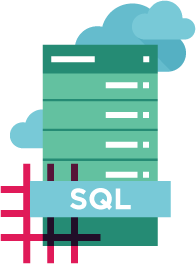## SQL Support Out-of-the-box

Transfer Data between Excel, SQL and GridViews by opening spreadsheets as System.Data.DataSet and System.Data.DataTable objects.## Modify Excel Data

Edit Microsoft Excel formulas - recalculate every time a work sheet it changed. Quick and Easy WorkSheet [“A1:B10”] formatting. Merge and set Ranges, Columns and Rows.Set Font, Size, Background, Border, Alignment and Number format.## Easy to Build with Visual Studio for .NET Core Projects

IronXL puts Excel Tools in your own hands with intellisense support. Install with NuGet for Visual Studio or a manual DLL download free for .NET Core. You'll be set up in the blink of an eye.

###### Supports:
•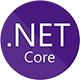•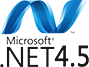•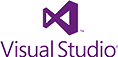•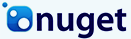•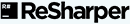•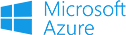## Licensing & Pricing for .NET Core

Free for development. Licenses from \$749.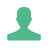### Project### Developer### Organization### Agency### SaaS## Excel Spreadsheet Tutorials for .NET Core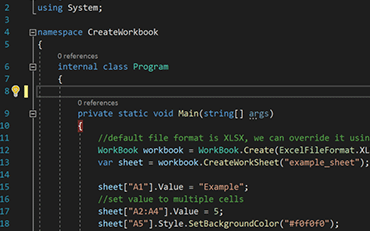C# Excel ASP.NET### How to Create Excel Files in .NET Core

See How Jonas uses IronXL to generate Excel Files without using Office Interop...

View Jonas' Excel File Generation Tutorial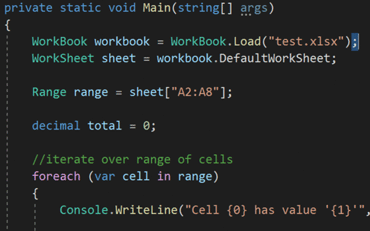C# Excel XLS### Open and Edit Excel Files in C# or VB.NET

Elijah shares a no-nonsense approach to reading Excel data into C# Applications...

See Elijah's Excel Reading & Editing Tutorial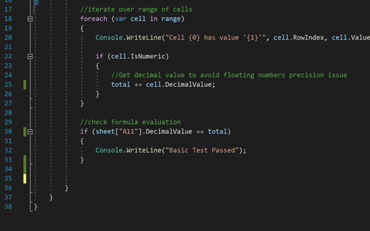C# Excel Data### How to Read Excel Files into .NET Apps.

Rebecca's code walkthrough for reading Excel data into a .NET application...

### Accounting and Finance Systems

• # Receipts
• # Reporting
• # Invoice Printing• # Documentation
• # Ordering & Labelling
• # Paper Replacement### Enterprise Content Management

• # Content Production
• # Document Management
• # Content Distribution### Data and Reporting Applications

• # Performance Tracking
• # Trend Mapping
• # Reports### Thousands of corporations, governments, SMEs and developers alike trust Iron software products.

Iron's team have over 10 years experience in the .NET software component market.